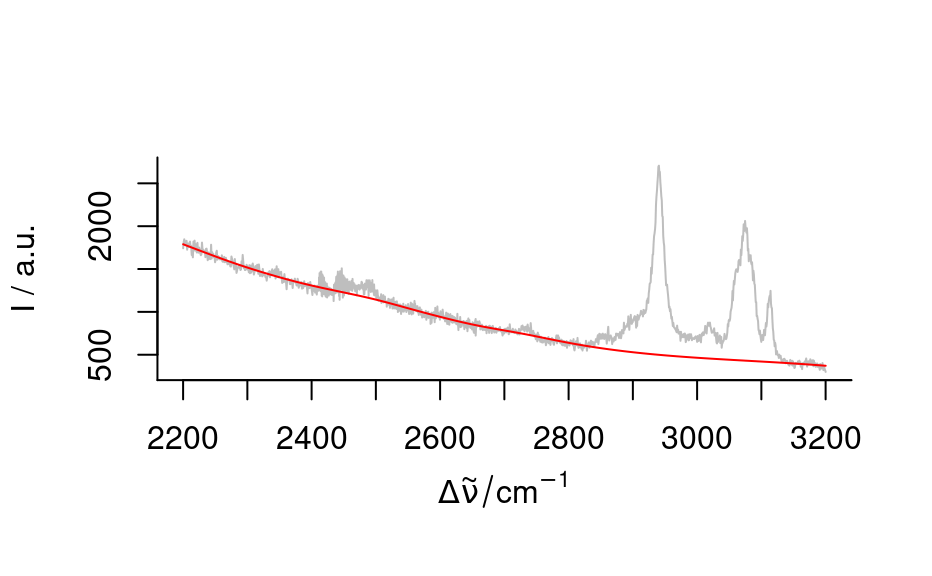Spectral smoothing by splines.

spc_smooth_spline(spc, newx = wl(spc), ...)

Arguments

spc

hyperSpec object

newx

wavelength axis to interpolate on

...

further parameters handed to stats::smooth.spline()

Value

hyperSpec object containing smoothed spectra

Note

This function is still experimental

Claudia Beleites

Examples

p <- paracetamol[, , 2200 ~ max]
plot(p, col = "gray")
smooth <- spc_smooth_spline(p[, , c(2200 ~ 2400, 2500 ~ 2825, 3150 ~ max)],
wl(paracetamol[, , 2200 ~ max]),
df = 4, spar = 1
)
plot(smooth, col = "red", add = TRUE)plot(p - smooth)